# JEE and NEET Important Questions Chapter Solutions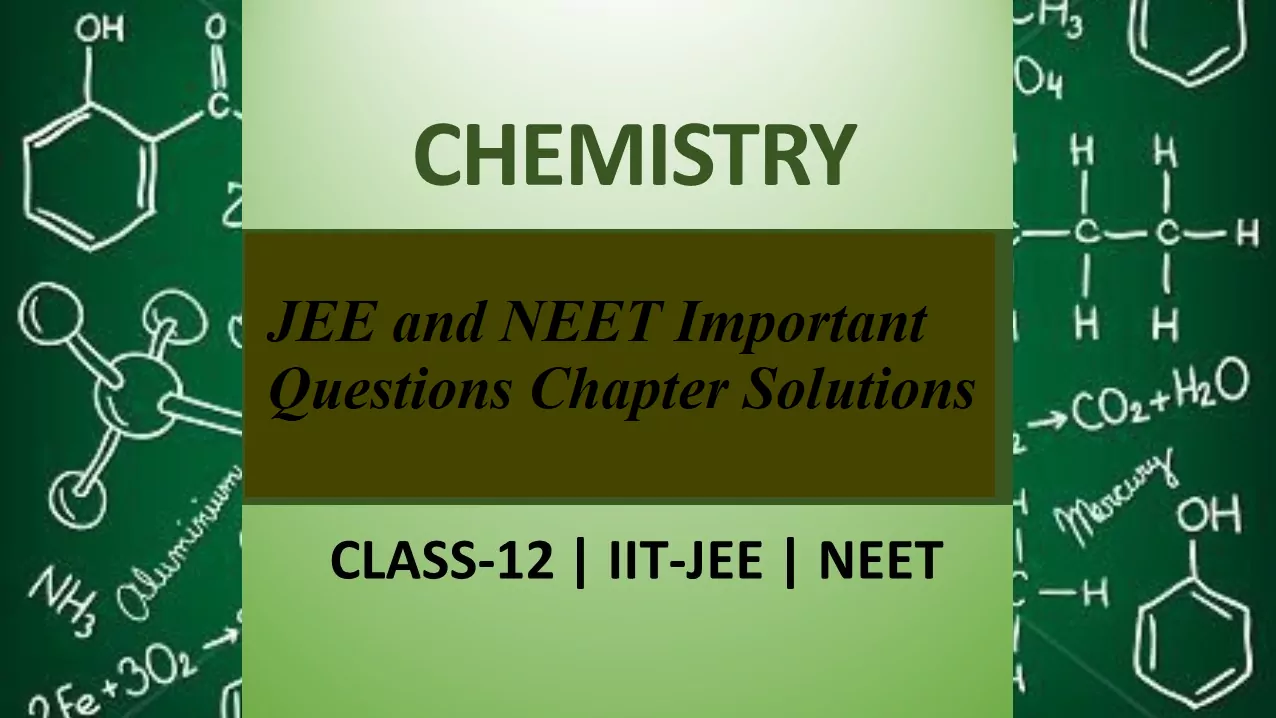# JEE and NEET Important Questions Chapter Solutions

Que 1. Which of the following units is useful in relating the concentration of a solution with its vapour pressure?
(i) Mole fraction                                                        (ii) Parts per million
(iii) Mass percentage                                                 (iv) Molality
Ans 1. (i)
Mole fraction is useful in relating vapour pressure with the concentration of the solution. According to Raoult’s law, the partial vapour pressure of each component in the solution is directly proportional to its mole fraction

Que 2. On dissolving sugar in water at room temperature solution feels cool to touch. Under which of the following cases dissolution of sugar will be most rapid?
(i) Sugar crystals in cold water.
(ii) Sugar crystals in hot water.
(iii) Powdered sugar in cold water.
(iv) Powdered sugar in hot water.
Ans 2. (iv)
Since the solution is cool to touch, the dissolution is endothermic. Therefore, high temperature will favour dissolution. Further, powdered sugar has a large surface area and is favourable for dissolution.

Que 3. What is the mole fraction of the solute in a 1.00 m aqueous solution?
(a) 1.770                                        (b) 0.0354
(c) 0.0177                                         (d) 0.177
Ans 3. (c)
1 molal aqueous solution means 1 mole of solute is present in 1000 g of water.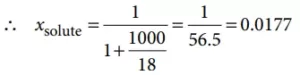Que 4. At equilibrium the rate of dissolution of a solid solute in a volatile liquid solvent is __________.
(i) Less than the rate of crystallisation
(ii) Greater than the rate of crystallisation
(iii) Equal to the rate of crystallisation
(iv) Zero
Ans 4. (iii)
At equilibrium, the rate of dissolution of solid solute is equal to the rate of crystallisation.

Que 5. How many grams of CH3OH should be added to water to prepare a 150 mL solution of 2 M CH3OH?
(a)  9.6 × 103                                      (b) 2.4 × 103
(c) 9.6                                                 (d) 2.4
Ans 5. (c)
Since the molecular mass of CH3OH is 32, therefore the quantity of CH3OH to prepare 150 mL solution of 2 M CH3OH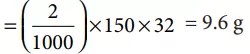JEE and NEET Important Questions Chapter Solutions

Que 6. A beaker contains a solution of the substance ‘A’. Precipitation of substance ‘A’ takes place when a small amount of ‘A’ is added to the solution. The solution is _________.
(i) Saturated
(ii) Supersaturated
(iii) Unsaturated
(iv) Concentrated
Ans 6. (ii)
When a small amount of solute is added to its solution and it does not dissolve and get precipitated then this solution is a supersaturated solution.

Que 7. The maximum amount of a solid solute that can be dissolved in a specified amount of a given liquid solvent does not depend upon ________.
(i) Temperature
(ii) Nature of solute
(iii) Pressure
(iv) Nature of solvent
Ans 7. (iii)
The maximum amount of solid that can be dissolved in a specified amount of a given solvent does not depend upon pressure. This is because solid and liquid are highly incompressible and practically remain unaffected by the change in pressure.

Que 8. The vapour pressure of two liquids P and Q are 80 and 60 torr, respectively. The total vapour pressure of solution obtained by mixing 3 moles of P and 2 mol of Q would be
(a) 72 torr                                           (b) 140 torr
(c)  68 torr                                          (d) 20 torr
Ans 8. (c)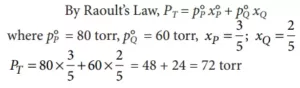Que 9. Low concentration of oxygen in the blood and tissues of people living at high altitude is due to ____________.
(i) Low temperature
(ii) Low atmospheric pressure
(iii) High atmospheric pressure
(iv) Both low temperature and high atmospheric pressure
Ans 9. (ii)
The low concentration of oxygen in the blood and tissues of people living at high altitudes is due to low atmospheric pressure. Because at high altitudes, the partial pressure of oxygen is less than at the ground level. This decreased atmospheric pressure causes the release of oxygen from the blood.

Que 10. Considering the formation, breaking and strength of hydrogen bond, predict which of the following mixtures will show a positive deviation from Raoult’s law?
(i) Methanol and acetone.
(ii) Chloroform and acetone.
(iii) Nitric acid and water.
(iv) Phenol and aniline.
Ans 10. (i)
On adding acetone, the extent of H-bonding decreases. Therefore, the solution shows a positive deviation.

JEE and NEET Important Questions Chapter Solutions

Que 11. Which condition is not satisfied by an ideal solution?
(a) ΔmixV = 0                                       (b) ΔmixS ≠ 0
(c) Obeyance to Raoult’s Law                        (d) ΔmixH = 0
Ans 11. (b)
For an ideal solution:
(i) Volume change (ΔV) on mixing should be zero.
(ii) Heat change (ΔH) on mixing should be zero.
(iii) Obeys Raoult’s law at every range of concentration.
(iv) Entropy change (ΔS) on mixing ≠ 0.

Que 12. Colligative properties depend on ____________.
(i) The nature of the solute particles dissolved in solution.
(ii) The number of solute particles in solution.
(iii) The physical properties of the solute particles dissolved in solution.
(iv) The nature of solvent particles.
Ans 12. (ii) The number of solute particles in solution

Que 13. Which of the following aqueous solutions should have the highest boiling point?
(i) 1.0 M NaOH
(ii) 1.0 M Na2SO4
(iii) 1.0 M NH4NO3
(iv) 1.0 M KNO3
Ans 13. (ii)
1.0 M Na2SO4 is correct because its molecular mass is the highest among all that’s why its boiling point will be the highest among the four.

Que 14. The unit of Ebulioscopic constant is _______________.
(i) K kg mol–1 or K (molality) –1
(ii) mol kg K–1 or K–1 (molality)
(iii) kg mol–1 K–1 or K–1 (molality) –1
(iv) K mol kg–1 or K (molality)
Ans 14. (i)

Que 15. In comparison to a 0.01 M solution of glucose, the depression in freezing point of a 0.01 M MgCl2 solution is _____________.
(i) The same
Ans 15. (iii)
0.01 M solution of glucose does not ionize while 0.01 M MgCl2 solution furnishes 3 ions (Mg2+ + 2Cl) in the solution, hence the value of the colligative property for MgCl2 solution is about 3 times.

JEE and NEET Important Questions Chapter Solutions

Que 16. A solution of sucrose (molar mass = 342 g mol–1) has been prepared by dissolving 68.5 g of sucrose in 1000 g of water. The freezing point of the solution obtained will be (Kf  for water = 1.86 K kg mol–1)
(a)  – 0.372 °C                                     (b) – 0.520 °C
(c)  + 0.372 °C                                     (d) – 0.570 °C
Ans 16. (c)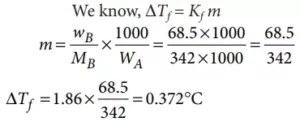Que 17.  An unripe mango placed in a concentrated salt solution to prepare pickle, shrivels because _____________.
(i) it gains water due to osmosis.
(ii) it loses water due to reverse osmosis.
(iii) it gains water due to reverse osmosis.
(iv) it loses water due to osmosis.
Ans 17. (iv)
When an unripe mango is placed in a concentrated salt solution to prepare pickle then mango lose water due to exo-osmosis and get shrivel

Que 18. At a given temperature, osmotic pressure of a concentrated solution of a substance _____________.
(i) is higher than that at a dilute solution.
(ii) is lower than that of a dilute solution.
(iii) Is the same as that of a dilute solution.
(iv) Cannot be compared with an osmotic pressure of the dilute solution.
Ans 18. (i)
According to the definition of osmotic pressure, we know that π=CRT. For concentrated solution, C has a higher value than a dilute solution. Hence as the concentration of solution increases, osmotic pressure will also increase.

Que 19. Which of the following statements is false?
(i) Two different solutions of sucrose of the same molality prepared in different solvents will have the same depression in freezing point.
(ii) The osmotic pressure of a solution is given by the equation Π = CRT (where C is the molarity of the solution).
(iii) Decreasing order of osmotic pressure for 0.01 M aqueous solutions of barium chloride, potassium chloride, acetic acid and sucrose is BaCl2 > KCl > CH3COOH > sucrose.
(iv) According to Raoult’s law, the vapour pressure exerted by a volatile component of a solution is directly proportional to its mole fraction in the solution.
Ans 19. (i)

Que 20. The values of Van’t Hoff factors for KCl, NaCl and K2SO4, respectively, are _____.
(i) 2, 2 and 2
(ii) 2, 2 and 3
(iii) 1, 1 and 2
(iv) 1, 1 and 1
Ans 20. (ii)

JEE and NEET Important Questions Chapter Solutions

Que 21. Which of the following statements is false?
(i) Units of atmospheric pressure and osmotic pressure are the same.
(ii) In reverse osmosis, solvent molecules move through a semipermeable membrane from a region of lower concentration of solute to a region of higher concentration.
(iii) The value of the molal depression constant depends on the nature of the solvent.
(iv) Relative lowering of vapour pressure, is a dimensionless quantity.
Ans 21. (ii)

Que 22. Of the following 0.10 m aqueous solutions, which one will exhibit the largest freezing point depression?
(a) KCl                                                            (b) C6H12O6
(c) Al2(SO4)3                                                  (d) K2SO4
Ans 22. (c)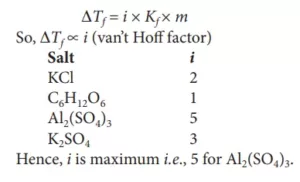Que 23. Value of Henry’s constant KH ____________.
(i) Increases with an increase in temperature.
(ii) Decreases with increase in temperature.
(iii) Remains constant.
(iv) First increases then decrease.
Ans 23. (i) Increases with increase in temperature

Que 24. The value of Henry’s constant KH is _____________.
(i) Greater for gases with higher solubility.
(ii) Greater for gases with lower solubility.
(iii) Constant for all gases.
(iv) Not related to the solubility of gases.
Ans 24. (ii) Greater for gases with lower solubility.

Que 25. If two liquids A and B form minimum boiling azeotrope at some specific composition then _______________.
(i) A–B interactions are stronger than those between A–A or B–B.
(ii) vapour pressure of solution increases because more molecules of liquids A and B can escape from the solution.
(iii) vapour pressure of solution decreases because less number of molecules of only one of the liquids escape from the solution.
(iv) A–B interactions are weaker than those between A–A or B–B.
Ans 25. (i) A–B interactions are stronger than those between A–A or B–B.

Que 26. We have three aqueous solutions of NaCl labelled as ‘A’, ‘B’ and ‘C’ with concentrations 0.1M, 0.01M and 0.001M, respectively. The value of van’t Hoff factor for these solutions will be in the order______.
(i) iA < iB < iC
(ii) iA > iB > iC
(iii) iA = iB = iC
(iv) iA < iB > iC
Ans 26. (iii)
The van’t Hoff factor for strong electrolyte is:
i = Total number of ions after dissociation/association/Total number of ions before dissociation/association.
For NaCl, the van’t Hoff factor is 2 as it dissociates into two ions i.e.
van’t Hoff factor is independent of the molality of solute added.
Thus, iA = iB = iC.

JEE and NEET Important Questions Chapter Solutions